# EE308 LAB1-2

 The Link Your Class https://bbs.csdn.net/forums/MUEE308FZU202201 The Link of Requirement of This Assignment https://bbs.csdn.net/topics/608734907 The Aim of This Assignment Output "keyword" statistics MU STU ID and FZU STU ID 20122772_832001311

1.PSP Table

2.Problem-solving ideas

3.Design and implementation process

4.Code description

5.Test

6.Performance analysis

7.Summary

https://github.com/li123sq/EE308FZ_Software-Engineer

# 1.PSP Table

PSPESTIMATE TIME（MINUTE）REAL TIME（MINUTE）
Planning2035
· Estimate55
Development2030
· Analysis100200
· Design Spec00
· Design Review1015
· Coding Standard2530
· Design6080
· Coding600700
· Code Review1530
· Test6080
Reporting//
· Test Repor1515
· Size Measurement1015
· Postmortem & Process Improvement Plan3040
11101375

# 2.Problem-solving ideas

The original idea was for each word to be compared to 32 keywords, but this was obviously a brute force algorithm and was not implemented. Therefore, I asked for help from the search engine and input "c++ search keywords". I got more feedback that it was Trie tree, so I went to learn about this knowledge point. Later, I learned about AC automata through communication with my classmates.The AC automaton algorithm mainly relies on constructing a finite state machine (similar to adding a mismatch pointer to a trie tree). These additional mismatch Pointers allow for backoff when the string lookup fails (for example, if the Trie tree fails to match the word cat, but there is another word cart in the Trie tree, the mismatch pointer will point to the prefix ca) and turn to other branches of a prefix, avoiding repeated matching of the prefix and improving the efficiency of the algorithm.See function flow chart for details.

# 3.Design and implementation process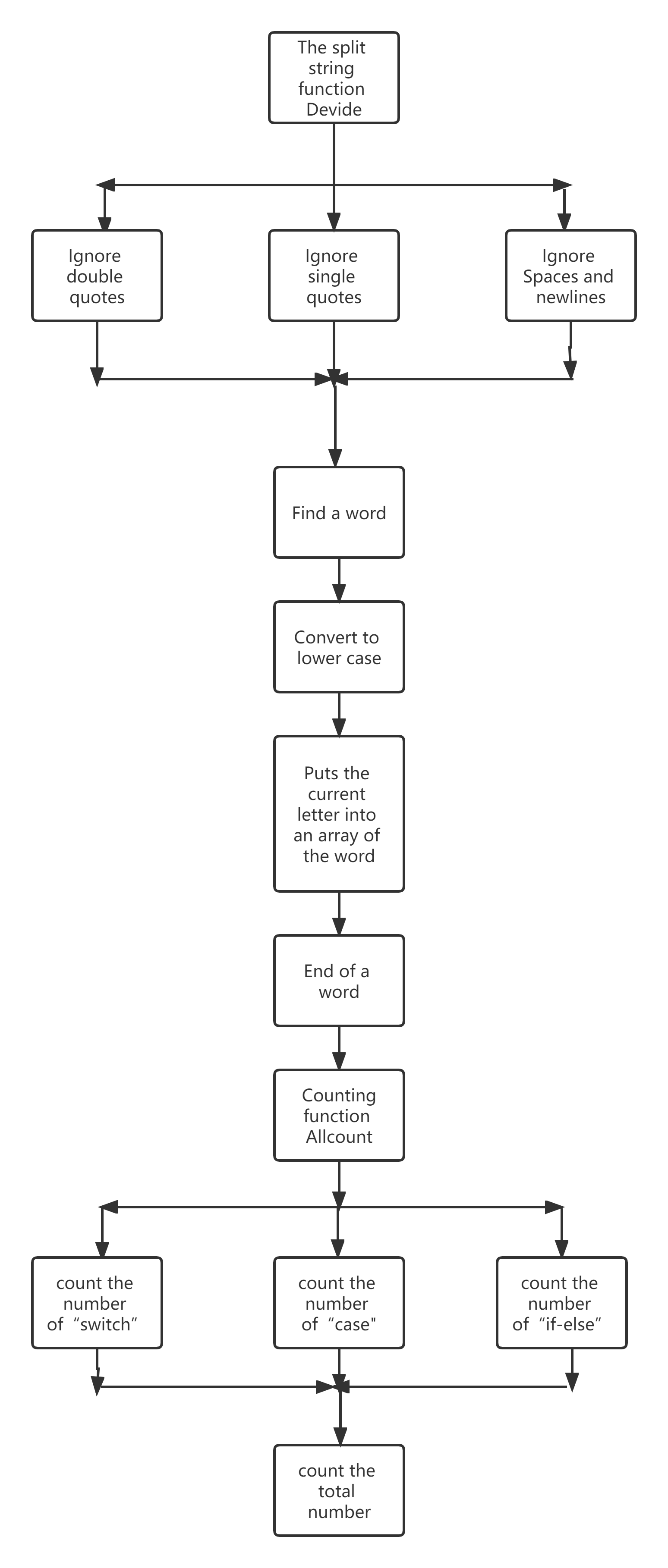# 4.Code description

The split string function Devide

``````void CodeTest::Devide()
{
while ((ch=fgetc(pfin)) != EOF)
{
state = fsm[state][ch];
switch (state)
{
case 5:
case 6:
case 0:
if (ch == '"')
{
ch = fgetc(pfin);
while (ch != '"')
{
ch = fgetc(pfin);
}
continue;
}
if (ch == 39)
{
ch = fgetc(pfin);
while (ch != 39)
{
ch = fgetc(pfin);
}
continue;
}
if (ch == ' ' || ch == 10)
{
continue;
}
if (ch == '{')
{
ifelse_stack.push(0);
}
if (ch == 125)
{
while (ifelse_stack.top() != 0)
{
ifelse_stack.pop();
}
ifelse_stack.pop();
}
if ((ch >= 'a' && ch <= 'z') || (ch >= 'A' && ch <= 'Z'))
{
danci = "";
while ((ch >= 'a' && ch <= 'z') || (ch >= 'A' && ch <= 'Z')
|| (ch == '_') || (ch == '/') || (ch == '.'))
{
if (ch >= 'A' && ch <= 'Z')
{
ch += 32;
}
danci += ch;
ch = fgetc(pfin);
}
Allcount(danci);
}
case 7:
state = 0;
break;
}
}
}``````

Counting function Allcount

``````void CodeTest::Allcount(string str)
{
if (str == "auto" || str == "break" || str == "case" || str == "char"
|| str == "const" || str == "continue" || str == "default" || str == "do"
|| str == "double" || str == "else" || str == "enum" || str == "extern"
|| str == "float" || str == "for" || str == "goto" || str == "if"
|| str == "int" || str == "long" || str == "register" || str == "return"
|| str == "short" || str == "signed" || str == "sizeof" || str == "static"
|| str == "struct"|| str == "switch" || str == "typedef" || str == "union"
|| str == "unsigned" || str == "void" || str == "volatile" || str == "while")
{
keyword++;
if (str == "switch")
{
flag = 1;
switch_num++;
}
if (str == "case" && flag == 1)
{
switch_else_num[switch_num]++;
}
if (str == "default" && flag == 1)
{
flag = 0;
}
if (str == "if")
{
ifelse_stack.push(-1);
ifelse_stack.push(1);
}
if (str == "else")
{
flag2 = 0;
flag3 = 0;
while (ch == ' ' || ch == 10)
{
ch = fgetc(pfin);
}
if (ch == 'i')
{
mark = "";
mark += ch;
ch = fgetc(pfin);
mark += ch;
if (mark == "if")
{
keyword++;
ifelse_stack.push(1);
flag2 = 1;
}
}
else if (ch == 123)
{
flag3 = 1;
}
if (flag2 == 0)
{
int ifelse_stack_count = 0;
while (ifelse_stack.top() > 0 && !ifelse_stack.empty())
{
ifelse_stack_count += ifelse_stack.top();
ifelse_stack.pop();
}
if (ifelse_stack_count > 1)
{
if_elseif_else_count++;
}
else if (ifelse_stack_count == 1)
{
if_else_count++;
}
if (flag3 == 1)
{
ifelse_stack.push(0);
}
}
}
}
}``````

main function

``````int main()
{
CodeTest *t = new CodeTest();
int level;
char filename;
cout<<"path：";
gets(filename);
cout<<"level：";
cin>>level;
if ((pfin=fopen(filename,"r"))==NULL)
{
cout << "File opening error";
return 0;
}
t->Delete(fsm);
t->Devide();
fclose(pfin);
t->print(level);
return 0;
}``````

# 5.Test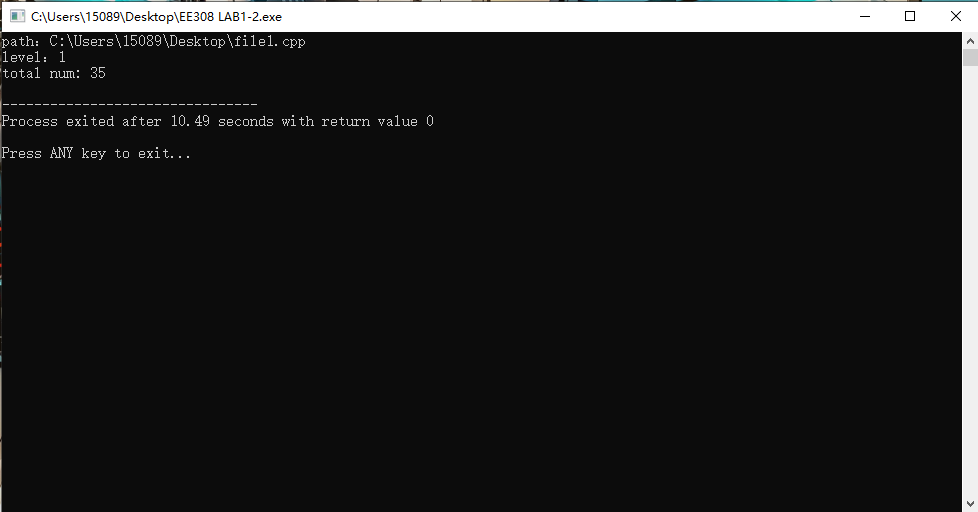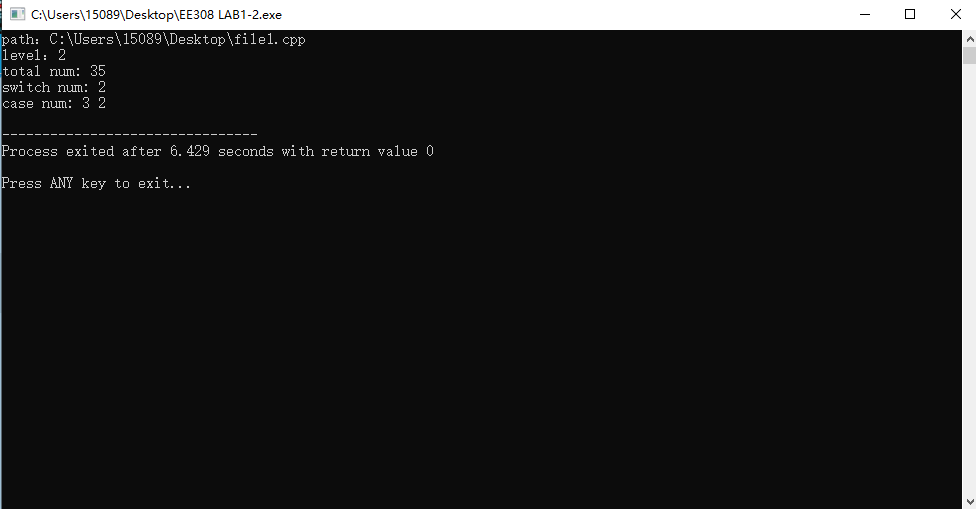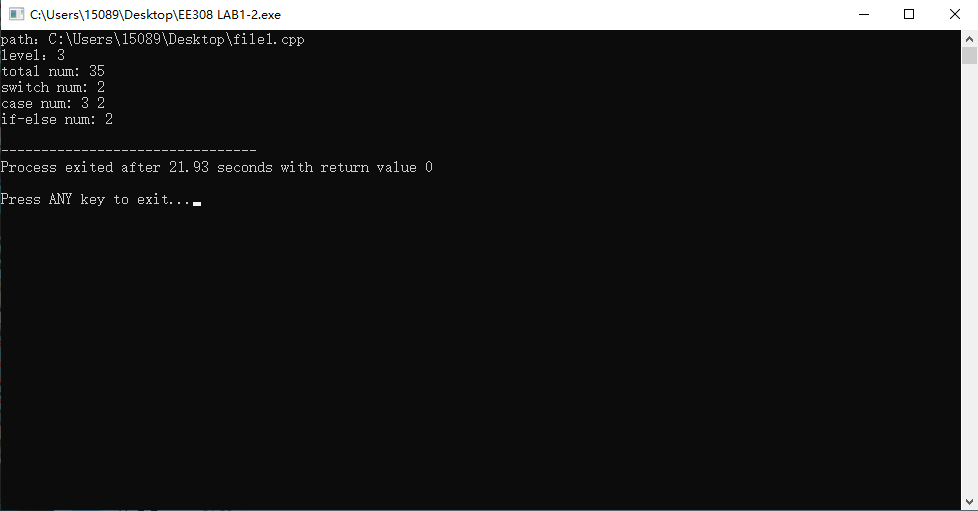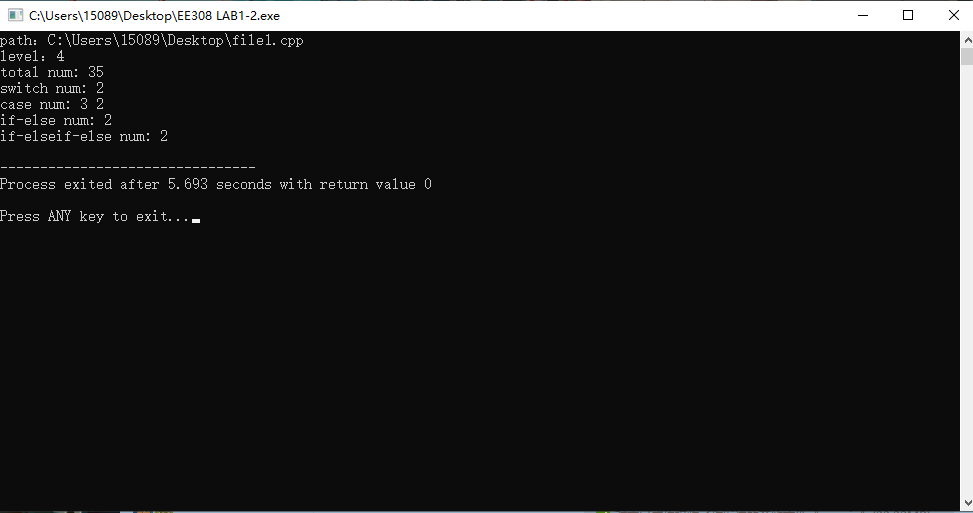# 6.Performance analysis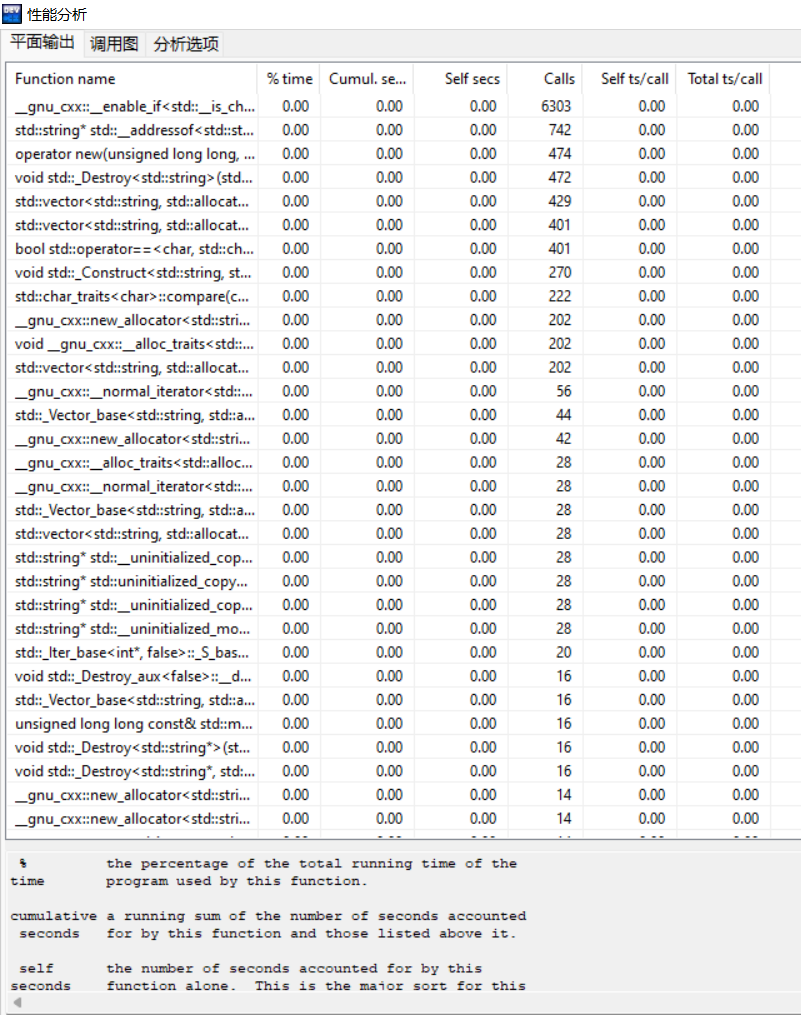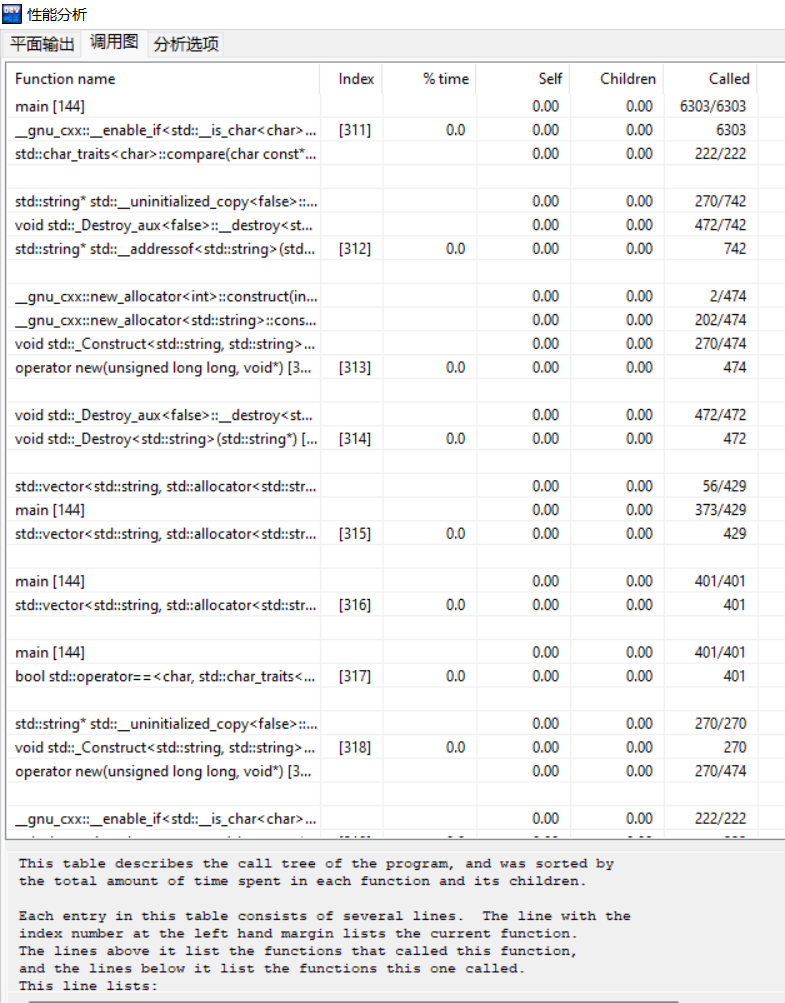# 7.Summary

I think this experiment is very difficult for me. So this time my code is written on the basis of my discussion with my classmates. But this experiment also strengthened my ability to write code.

...全文
109 回复 打赏 收藏 举报JOS 内核设计代码
JOS教学操作系统LAB1-2的源代码实现，完整得完成了实验内容.EE308 Lab1-2
EE308 Lab1-2。lab1-2
lab1-2 extract keywordsEE308 Lab1-1
EE308 Lab1-1EE308 Lab2
EE308 Lab2

286